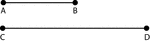Segments, 2 Line

2 line segments, AB and CD. AB is half the length of CD.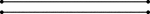Segments, 2 Parallel Line

2 parallel horizontal line segments.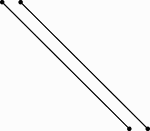Segments, 2 Parallel Line

2 slanted parallel line segments.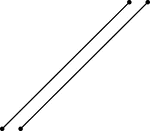Segments, 2 Parallel Line

2 slanted parallel line segments.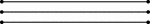Segments, 3 Parallel Line

3 parallel horizontal line segments.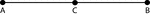Segment, 3 Points on a Line

Illustration of line segment AB, which is made up of line segments AC and CB. C is considered the midpoint…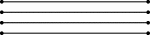Segments, 4 Parallel Line

4 parallel horizontal line segments.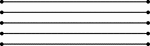Segments, 5 Parallel Line

5 parallel horizontal line segments.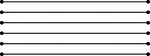Segments, 6 Parallel Line

6 parallel horizontal line segments.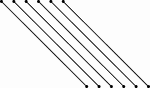Segments, 6 Parallel Line

6 slanted parallel line segments.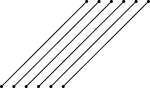Segments, 6 Parallel Line

6 slanted parallel line segments.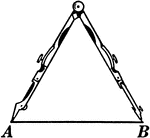Compass and Line

Illustration of a compass with one leg at point A on the line segment and the other at Point B.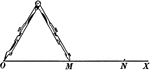Compass and Line

Illustration of a compass with one leg at point O on the line segment and the other at Point M.Segment, Horizontal Line

Illustration of a horizontal line segment. A line segment is a section of a line with definite endpoints.Segment, Horizontal Line

Illustration of a horizontal line segment. A line segment is a section of a line with definite endpoints.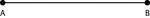Segment, Horizontal Line

Illustration of a horizontal line segment. A line segment is a section of a line with definite endpoints.…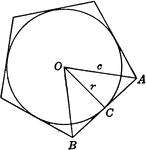Regular Pentagon With Circle Inscribed

Illustration showing a circle inscribed in a regular pentagon. Or, a regular pentagon circumscribed…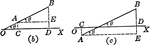Projection of a Line Segment

CD is the projection of AB upon OX. In each case AE=CD and AE=ABcos theta.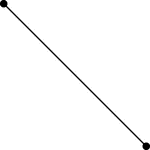Segment, Slanted Line

Illustration of a slanted line segment. A line segment is a section of a line with definite endpoints.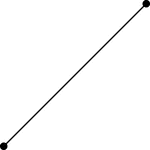Segment, Slanted Line

Illustration of a slanted line segment. A line segment is a section of a line with definite endpoints.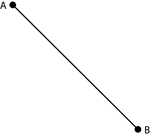Segment, Slanted Line

Illustration of a slanted line segment. A line segment is a section of a line with definite endpoints.…Segment, Slanted Line

Illustration of a slanted line segment. A line segment is a section of a line with definite endpoints.…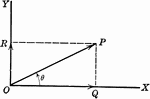Vector

OP is a line representing a vector (directed quantity)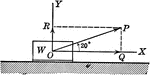Vector Showing Force at Angle of 20 degrees

OP is a vector (directed quantity) showing the force by a weight acting on it at an angle of 20 degrees.Segment, Vertical Line

Illustration of a vertical line segment. A line segment is a section of a line with definite endpoints.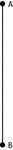Segment, Vertical Line

Illustration of a vertical line segment. A line segment is a section of a line with definite endpoints.…